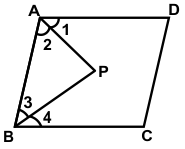# CREST Mathematics Olympiad Class 9 Previous Year Papers

## Syllabus:

Section 1: Number Systems, Polynomials, Coordinate Geometry, Linear Equations in Two Variables, Introduction to Euclid’s Geometry, Lines and Angles, Triangles, Quadrilaterals, Areas of Parallelograms and Triangles, Circles, Constructions, Heron’s Formula, Surface Areas and Volumes, Statistics, Probability.

Achievers Section: Higher Order Thinking Questions - Syllabus as per Section 1

 Q.1 The numerical expression 3/8 + (-5)/7 = -19/56 shows that:
 Q.2 If x = a(b - c), y = b(c - a) and z = c(a - b), then (x/a)3 + (y/b)3 + (z/c)3 = ?
 Q.3 Two years ago, the ratio of A's age to B's age at that time was 5:9. A's age three years ago was 13 years less than B's age six years ago. What is B's present age?
 Q.4 In measuring the sides of a rectangle, there is an excess of 5% on one side and 2% deficit on the other. Then the error percent in the area is:
 Q.5 In the given figure, AP and BP are angle bisectors of ∠A and ∠B, respectively which meets at P on the parallelogram ABCD. Then 2∠APB =  ?Q.6 Which of the following relationship is correct?
 Q.7 The ratio of the number of students in two classrooms, C1 and C2, is 2:3. It is observed that after shifting ten students from C1 to C2, the ratio is 3:7. Further, how many students have to be shifted from C2 to C1 for the new ratio to become 9:11?
 Q.8 From four corners of a square sheet of side 4 cm, four pieces, each in the shape of an arc of a circle with radius 2 cm are cut out. The area of the remaining portion is:
 Q.9 The circum-centre of the triangle formed by points O(0, 0), A(6, 0) and B(0, 6) is ___________.
 Q.10 It is known that if x + y = 10, then x + y + z = 10 + z. The Euclid's axiom that illustrates this statement is:

Answers to Previous Year Questions from CREST Olympiads:

 Q.1 a Q.2 a Q.3 a Q.4 c Q.5 a Q.6 a Q.7 b Q.8 b Q.9 a Q.10 b

Previous Year PDF of CREST Mathematics Olympiad for Class 9: